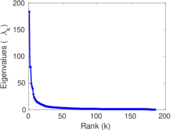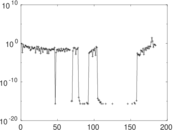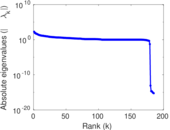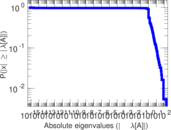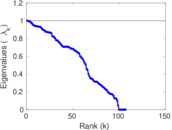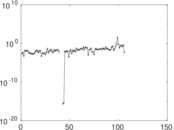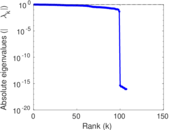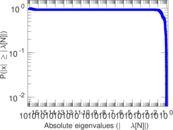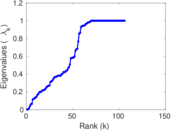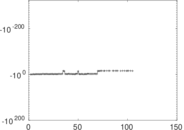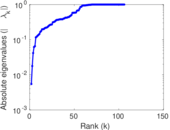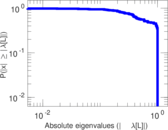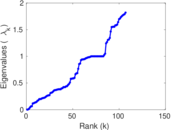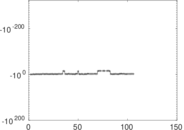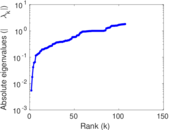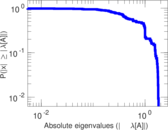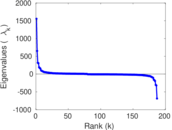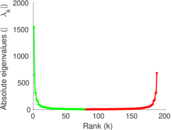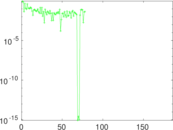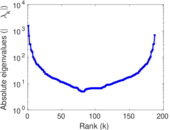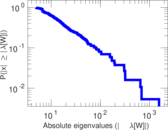# Wiktionary edits (gv)

This is the bipartite edit network of the Manx Wiktionary. It contains users and pages from the Manx Wiktionary, connected by edit events. Each edge represents an edit. The dataset includes the timestamp of each edit.

 Code `mgv` Internal name `edit-gvwiktionary` Name Wiktionary edits (gv) Data source http://dumps.wikimedia.org/ AvailabilityDataset is available for download Consistency checkDataset passed all tests Category Authorship network Dataset timestamp 2017-10-20 Node meaning User, article Edge meaning Edit Network formatBipartite, undirected Edge typeUnweighted, multiple edges Temporal dataEdges are annotated with timestamps

## Statistics

 Size n = 1,530 Left size n1 = 187 Right size n2 = 1,343 Volume m = 8,287 Unique edge count m̿ = 3,942 Wedge count s = 573,548 Claw count z = 86,837,377 Cross count x = 11,734,793,431 Square count q = 590,562 4-Tour count T4 = 7,026,880 Maximum degree dmax = 2,507 Maximum left degree d1max = 2,507 Maximum right degree d2max = 114 Average degree d = 10.832 7 Average left degree d1 = 44.315 5 Average right degree d2 = 6.170 51 Fill p = 0.015 696 4 Average edge multiplicity m̃ = 2.102 23 Size of LCC N = 1,250 Diameter δ = 17 50-Percentile effective diameter δ0.5 = 3.929 47 90-Percentile effective diameter δ0.9 = 7.973 63 Median distance δM = 4 Mean distance δm = 5.055 36 Gini coefficient G = 0.769 181 Balanced inequality ratio P = 0.202 425 Left balanced inequality ratio P1 = 0.090 985 9 Right balanced inequality ratio P2 = 0.253 892 Relative edge distribution entropy Her = 0.783 765 Power law exponent γ = 2.299 62 Tail power law exponent γt = 1.821 00 Tail power law exponent with p γ3 = 1.821 00 p-value p = 0.000 00 Left tail power law exponent with p γ3,1 = 1.651 00 Left p-value p1 = 0.063 000 0 Right tail power law exponent with p γ3,2 = 8.991 00 Right p-value p2 = 0.792 000 Degree assortativity ρ = +0.008 719 94 Degree assortativity p-value pρ = 0.584 158 Spectral norm α = 183.634 Algebraic connectivity a = 0.005 452 47 Spectral separation |λ1[A] / λ2[A]| = 2.290 05 Controllability C = 1,169 Relative controllability Cr = 0.765 553

## Plots

### Fruchterman–Reingold graph drawing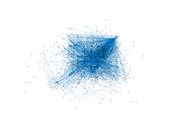### Degree distribution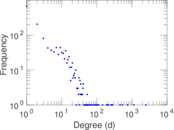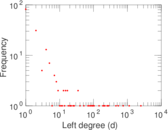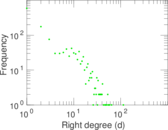### Cumulative degree distribution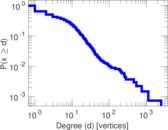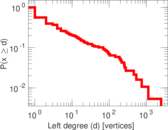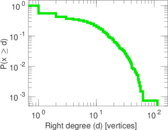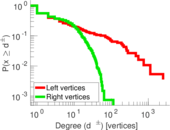### Lorenz curve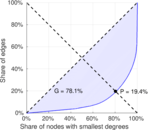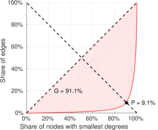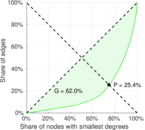### Spectral distribution of the adjacency matrix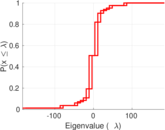### Spectral distribution of the normalized adjacency matrix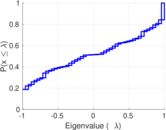### Spectral distribution of the Laplacian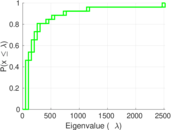### Spectral graph drawing based on the adjacency matrix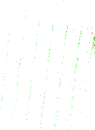### Spectral graph drawing based on the Laplacian### Spectral graph drawing based on the normalized adjacency matrix### Degree assortativity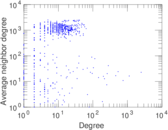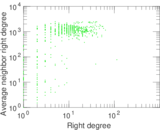### Zipf plot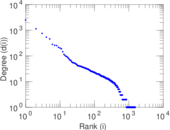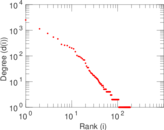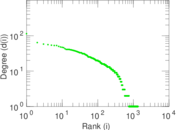### Hop distribution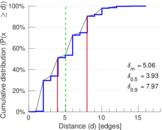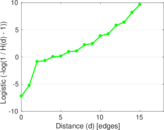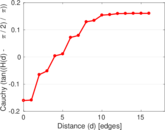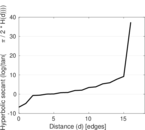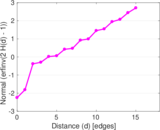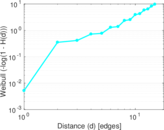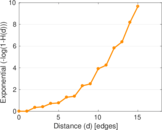### Double Laplacian graph drawing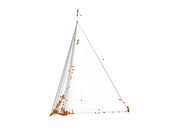### Delaunay graph drawing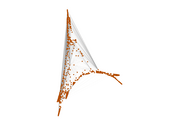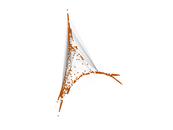### Edge weight/multiplicity distribution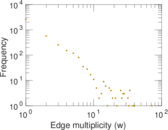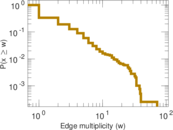### Temporal distribution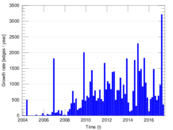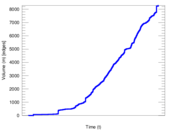### Temporal hop distribution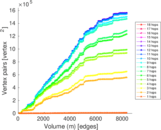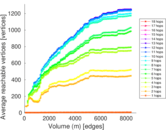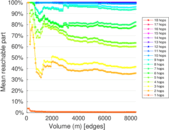### Diameter/density evolution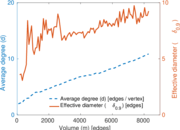### Matrix decompositions plots